CAT  >  Sample IIFT Data Interpretation Quiz

# Sample IIFT Data Interpretation Quiz

Test Description

## 18 Questions MCQ Test IIFT Mock Test Series | Sample IIFT Data Interpretation Quiz

Sample IIFT Data Interpretation Quiz for CAT 2022 is part of IIFT Mock Test Series preparation. The Sample IIFT Data Interpretation Quiz questions and answers have been prepared according to the CAT exam syllabus.The Sample IIFT Data Interpretation Quiz MCQs are made for CAT 2022 Exam. Find important definitions, questions, notes, meanings, examples, exercises, MCQs and online tests for Sample IIFT Data Interpretation Quiz below.
Solutions of Sample IIFT Data Interpretation Quiz questions in English are available as part of our IIFT Mock Test Series for CAT & Sample IIFT Data Interpretation Quiz solutions in Hindi for IIFT Mock Test Series course. Download more important topics, notes, lectures and mock test series for CAT Exam by signing up for free. Attempt Sample IIFT Data Interpretation Quiz | 18 questions in 20 minutes | Mock test for CAT preparation | Free important questions MCQ to study IIFT Mock Test Series for CAT Exam | Download free PDF with solutions
 1 Crore+ students have signed up on EduRev. Have you?
Sample IIFT Data Interpretation Quiz - Question 1

### Group Question Answer the following question based on the information given below. The following bar chart gives the percentage growth in the number of households in the middle, upper middle and high income categories in the four regions for the period from 1987-88 to 1994-95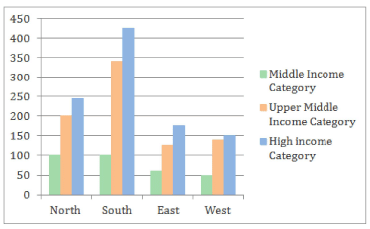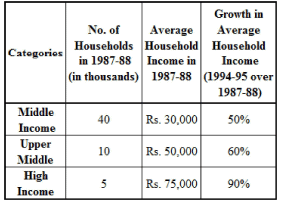Additional directions for the last two questions of this set: If the same data as the above were used, with the additional information that the numbers of households in each category were equally distributed in all the regions then Q. Which region showed the highest growth rate in the number of households in all the income categories for the period?

Detailed Solution for Sample IIFT Data Interpretation Quiz - Question 1

It can be seen from the graph that the southern region showed the highest growth in number of household in all the income categories for the period.

Sample IIFT Data Interpretation Quiz - Question 2

### The following bar chart gives the percentage growth in the number of households in the middle, upper middle and high income categories in the four regions for the period from 1987-88 to 1994-95Additional directions for the last two questions of this set: If the same data as the above were used, with the additional information that the numbers of households in each category were equally distributed in all the regions then Q. What was the total household income in the Northern Region for the upper middle class?

Detailed Solution for Sample IIFT Data Interpretation Quiz - Question 2

Using the number of households and the average income per household for the upper middle class, the total income for the upper middle class can be found.
However, the region wise distribution of upper middle class households is not known.
Hence, the total household income in the Northern region for the upper middle class cannot be found. Hence, option 4.

Sample IIFT Data Interpretation Quiz - Question 3

### The following bar chart gives the percentage growth in the number of households in the middle, upper middle and high income categories in the four regions for the period from 1987-88 to 1994-95Additional directions for the last two questions of this set: If the same data as the above were used, with the additional information that the numbers of households in each category were equally distributed in all the regions then Q.What is the percentage increase in the total number of households for the Northern Region (upper middle) over the given period?

Detailed Solution for Sample IIFT Data Interpretation Quiz - Question 3

This question can be answered by direct observation.
From the bar chart, the percentage increase in the total number of households for the Northern region (upper middles class) is 200%.
Hence, option 2.

Sample IIFT Data Interpretation Quiz - Question 4

The following bar chart gives the percentage growth in the number of households in the middle, upper middle and high income categories in the four regions for the period from 1987-88 to 1994-95Additional directions for the last two questions of this set: If the same data as the above were used, with the additional information that the numbers of households in each category were equally distributed in all the regions then

Q. What was the average income of the high-income group in 1987-88?

Detailed Solution for Sample IIFT Data Interpretation Quiz - Question 4

From the table, the average income of the high income group in 1987-88 was Rs. 75,000.
Hence, option 1.

Sample IIFT Data Interpretation Quiz - Question 5

The following bar chart gives the percentage growth in the number of households in the middle, upper middle and high income categories in the four regions for the period from 1987-88 to 1994-95Additional directions for the last two questions of this set: If the same data as the above were used, with the additional information that the numbers of households in each category were equally distributed in all the regions then

Q. The ratio of total income for the high income category to the upper middle class increased by how much  percent in the given period?

Detailed Solution for Sample IIFT Data Interpretation Quiz - Question 5

In 1987-88:
The total income for the high income category households = 5000 x 75000 = Rs. 37,50,00,000 The total income for the upper middle class households = 10000 x 50000 = Rs. 50,00,00,000 Thus, the required ratio for 1987-88 = 375000000/500000000 = 3 : 4 = 0.75 It is given that the number of households in each category is equally distributed in all the regions.
Thus, there were 5000/4 = 1250 high income household in each region in 1987-88.
Similarly, there were 10000/4 = 2500 upper middle class households in each region in 1987-88.
In the year 1994-95:

The percentage growth in high income households in the four regions is 245%, 425%, 175% and 150% Thus, the number of high income households throughout the country in 1994-95 is: 1250 x (3.45 + 5.25 + 2.75 + 2.5) i.e. 1250 x 13.95 i.e 17438 households (approx) The percentage growth in upper middle class households in the four regions is 200%, 340%, 120% and 140%
Thus, the number of upper middle class households throughout the country in 1994-95 is: 2500 x (3 + 4.4 + 2.2 + 2.4) i.e. 2500 x 12 i.e 30000 households (approx). Therefore Total income for the high-income households = 17438 x 1.90 x 75000 = Rs. 2,48,49,15,000. Total income for upper middle class = 30000 x 1.6 x 50000 = Rs. 2,40,00,00,000 Thus, the required ratio for 1994-95 = 2484915000/2400000000 = 1.035 Thus, the ratio has increased in absolute terms by 1.035 - 0.75 = 0.285 Hence, the required percentage increase = (0.285/0.75) x 100
= 38%
The closest option is 36%.
Hence, option 2.

Sample IIFT Data Interpretation Quiz - Question 6

The following bar chart gives the percentage growth in the number of households in the middle, upper middle and high income categories in the four regions for the period from 1987-88 to 1994-95Additional directions for the last two questions of this set: If the same data as the above were used, with the additional information that the numbers of households in each category were equally distributed in all the regions then

Q. The average income for the Northern region in 1987-88, was _____.

Detailed Solution for Sample IIFT Data Interpretation Quiz - Question 6

The total income for the Northern region in 1987-88 = [(40000 x 30000) + (10000 x 50000) + (5000 x 75000)]/4 = (1200000000 + 500000000 + 375000000)/4
= 2075000000/4
= Rs. 51,87,50,000 The total number of household in the Norther region in 1987-88 = (40000 + 10000 + 5000)/4.

= 55000/4
=13750
Thus, the average income for the Northern region in 1987-88 = 518750000/13750
= Rs. 37,727 (approx) Hence, option 1.

Sample IIFT Data Interpretation Quiz - Question 7

Group Question

Answer the following question based on the information given below.
Owing to a global economic slowdown the company Testa Ltd. has decided to review its employee compensation structure in order to cut costs. In the existing structure all 100 employees are paid a Fixed Salary (FS) equal to 10000 and a Variable Salary (VS), where the Variable Salary depends upon the performance of the company and is decided as a percentage of the Fixed Salary.

Employee salary = FS + VS The company is thinking of changing it into a variable pay structure where different groups of employees are paid as shown in the table below.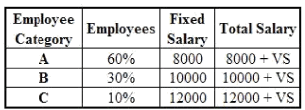Where the Variable Salary depends on the company performance and is calculated as a percentage of Fixed salary.

Q. The company does not perform satisfactorily and decides on a variable salary = 10% of fixed salary. What  is the percentage gain/loss of the company if it uses the new structure with respect to the existing compensation structure?

Detailed Solution for Sample IIFT Data Interpretation Quiz - Question 7

As there are 100 employees and VS = 10% of FS In the existing structure, the total salary would be = 100 x (10000 + 10% of 10000) = Rs. 1100000
In the proposed new structure, total salary = 60 x (8000 + 10% of 8000) + 30 x (10000 + 10% of 10000) + 10 x (12000 + 10% of 12000)
= Rs. 990000 Difference in total salary = 1100000 - 990000 = Rs. 110000. The company saves Rs. 110000 if it uses the new compensation structure. Percentage gain = (110000/1100000) x 100 = 10%

Hence, option 3.

Sample IIFT Data Interpretation Quiz - Question 8

Owing to a global economic slowdown the company Testa Ltd. has decided to review its employee compensation structure in order to cut costs. In the existing structure all 100 employees are paid a Fixed Salary (FS) equal to 10000 and a Variable Salary (VS), where the Variable Salary depends upon the performance of the company and is decided as a percentage of the Fixed Salary.

Employee salary = FS + VS The company is thinking of changing it into a variable pay structure where different groups of employees are paid as shown in the table below.Where the Variable Salary depends on the company performance and is calculated as a percentage of Fixed salary.

Q. If the variable salary is decided as 15% of the fixed salary in the existing compensation structure, what should be the percentage variable pay in the new compensation structure such that there is no difference in total employee compensation between both the structures?

Detailed Solution for Sample IIFT Data Interpretation Quiz - Question 8

YS = 15% of FS Total employee salary in the existing compensation structure = 100 x (10000 + 15% of 10000)  = Rs. 1150000

Let the  variable salary be x% of the fixed salary in the new compensation structure. Total salary in the new compensation structure = 60 x (8000 + x% of 8000) + 30 x (10000 + x% of 10000) + 10 x (12000 + x% of 12000)
= Rs. (900000 + 9000x)

For there to be no difference between the two compensation structures, 1150000 = 900000 + 9000x.
x = (250/9) % = 27.78% Hence, option 4.

Sample IIFT Data Interpretation Quiz - Question 9

Owing to a global economic slowdown the company Testa Ltd. has decided to review its employee compensation structure in order to cut costs. In the existing structure all 100 employees are paid a Fixed Salary (FS) equal to 10000 and a Variable Salary (VS), where the Variable Salary depends upon the performance of the company and is decided as a percentage of the Fixed Salary.

Employee salary = FS + VS The company is thinking of changing it into a variable pay structure where different groups of employees are paid as shown in the table below.Where the Variable Salary depends on the company performance and is calculated as a percentage of Fixed salary.

Q. The company is not satisfied with the cost savings from the new compensation structure and decides to retrench 9 employees from employee categories of A and B such that employees retrenched from category A is twice those retrenched from category B. The variable pay for the new compensation is declared to be 10%. What should be the percentage variable pay in the old compensation structure such that there is no difference between the two?

Detailed Solution for Sample IIFT Data Interpretation Quiz - Question 9

As the company retrenches 9 employees the total employees now left are 91.

Let the employees retrenched from category B be y.
Employees retrenched from category A = 2y
y + 2y = 9.

y = 3. The employees in category A, B and C are 54, 27 and 10 respectively.
Total employee compensation in the new structure = 54 x (8000 + 10% of 8000) + 27 x (10000 + 10% of 10000) + 10 x (12000 + 10% of 12000).

Let the variable salary in the old compensation structure be z% of the fixed salary Total employee compensation in the old structure = 91 x (10000 + z% of 10000) = Rs. (910000 + 9100z) For there to be no difference in the two compensation structures, 904200 = 910000 + 9100z, which is not possible So, the total compensation of both the structures can never be same.
Hence, option 4.

Sample IIFT Data Interpretation Quiz - Question 10

Owing to a global economic slowdown the company Testa Ltd. has decided to review its employee compensation structure in order to cut costs. In the existing structure all 100 employees are paid a Fixed Salary (FS) equal to 10000 and a Variable Salary (VS), where the Variable Salary depends upon the performance of the company and is decided as a percentage of the Fixed Salary.

Employee salary = FS + VS The company is thinking of changing it into a variable pay structure where different groups of employees are paid as shown in the table below.Where the Variable Salary depends on the company performance and is calculated as a percentage of Fixed salary.

Q. If the total variable salary component of category A employees is equal to the total salary of category C employees, in the new system, then what percentage of the fixed salary is the variable salary?

Detailed Solution for Sample IIFT Data Interpretation Quiz - Question 10

Let the variable salary be x% of the fixed salary. Then, 60 * x% of 8000 = 10 *(12000 + x% of 12000). Therefore  4800x= 12000 + 1200x.
x= 33.33%
Hence, option 1

Sample IIFT Data Interpretation Quiz - Question 11

Group Question

Answer the following question based on the information given below.
Analyze the charts given below that show the energy consumption and energy production of XYZ country from various sources. The difference in production and consumption of energy from any source is bridged through external trade (i.e. export or import) of energy from the same source. All the values are given in Terawatt-hours (Twh).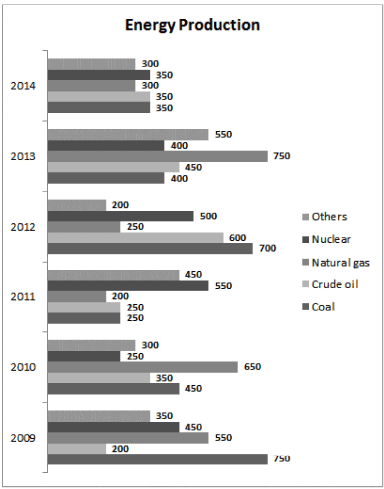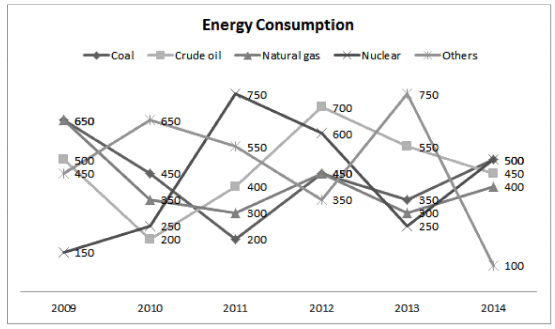Q. Which year registered the highest external trade in the energy  sector?

Detailed Solution for Sample IIFT Data Interpretation Quiz - Question 11

External trade = | Imports coal | + | Imports c rude oil I + I Imports Natural gas I + I Imports Nuclear I + I Imports others I

Imports = Consumption - Production In the equation above, exports have also been considered as imports i.e. a negative value of imports would actually mean an export.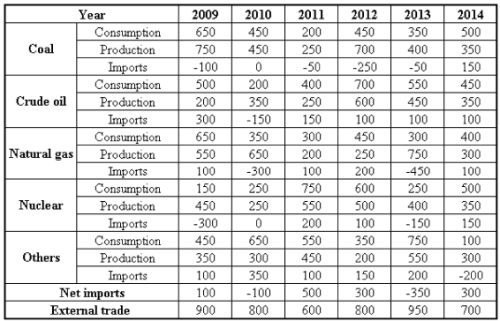From the table, the external trade is highest in the year 2013. Hence, option 4.

Sample IIFT Data Interpretation Quiz - Question 12

Analyze the charts given below that show the energy consumption and energy production of XYZ country from various sources. The difference in production and consumption of energy from any source is bridged through external trade (i.e. export or import) of energy from the same source. All the values are given in Terawatt-hours (Twh).Q. In which year are the net imports of all the categories taken together the lowest?

Detailed Solution for Sample IIFT Data Interpretation Quiz - Question 12

Consider the solution to the first question.
Among the given options, net imports are lowest in 2009. Hence, option 1.

Sample IIFT Data Interpretation Quiz - Question 13

Analyze the charts given below that show the energy consumption and energy production of XYZ country from various sources. The difference in production and consumption of energy from any source is bridged through external trade (i.e. export or import) of energy from the same source. All the values are given in Terawatt-hours (Twh).Q. Which of the following statement(s) is/are true?
I. In each year, net exports are always less than the external trade.
II. Coal is always exported.
III. Net import of energy from at least one source is nil from 2009- 2014.

Detailed Solution for Sample IIFT Data Interpretation Quiz - Question 13

Net exports = - net imports From the table, it is always less than the external trade.
Thus, statement I is true.
Import of coal in 2014 is 150TWh.
Thus, statement II is false.
Net import of nuclear energy = -300 + 0 + 200 + 100 -150 + 150 = 0
Thus, statement III is true.
Hence, option 3.

Sample IIFT Data Interpretation Quiz - Question 14

Group Question

Answer the following question based on the information given below.
Eight friends Raju, Ravi, Ramesh, Rahul, Arjun, Arvind, Anish and Atul are skilled at making Handicraft items. In order to make money using their skills, they decide to make a certain number of handicraft items and sell them at a Handicraft Expo. They decided to minimize time and maximize their productivity by forming two groups. The people whose names start with the letter ‘R’ form Group 1 and the people whose names start with the letter ‘A’ form Group 2. Each person from Group 1 forms a team each person from Group 2 to produce a certain number of handicraft items of a particular type, the details of which are given in the tables below.
Table 1 gives details of the type of handicraft item produced by each team.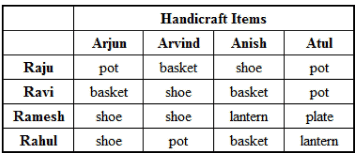Table 2 gives details of the number of handicraft items produced by each team.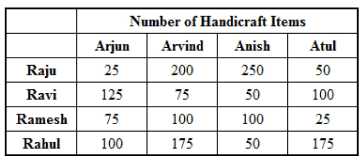Table 3 gives the cost price and selling price of one item of each type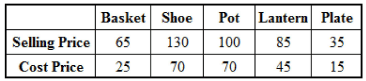The money (Cost Price and Profit) is shared according to the number of items each one has worked on. For example, if Rahul and Arvind made 175 pots, the total cost price and the profit made by selling those pots would be shared equally.

Q. Who has worked on the maximum number of handicraft items?

Detailed Solution for Sample IIFT Data Interpretation Quiz - Question 14

The number of handicraft items worked on by any person is directly given in table 2.
Raju, Rahul, Arvind and Anish have worked on 525, 500, 550 and 450 items respectively.
Thus, among these four people, Arvind has worked on the maximum number of handicraft items.
Hence, option 3.

Sample IIFT Data Interpretation Quiz - Question 15

Eight friends Raju, Ravi, Ramesh, Rahul, Arjun, Arvind, Anish and Atul are skilled at making Handicraft items. In order to make money using their skills, they decide to make a certain number of handicraft items and sell them at a Handicraft Expo. They decided to minimize time and maximize their productivity by forming two groups. The people whose names start with the letter ‘R’ form Group 1 and the people whose names start with the letter ‘A’ form Group 2. Each person from Group 1 forms a team each person from Group 2 to produce a certain number of handicraft items of a particular type, the details of which are given in the tables below.
Table 1 gives details of the type of handicraft item produced by each team.Table 2 gives details of the number of handicraft items produced by each team.Table 3 gives the cost price and selling price of one item of each typeThe money (Cost Price and Profit) is shared according to the number of items each one has worked on. For example, if Rahul and Arvind made 175 pots, the total cost price and the profit made by selling those pots would be shared equally.

Q. For how many teams was the total selling price more than Rs. 15,000?

Detailed Solution for Sample IIFT Data Interpretation Quiz - Question 15

The total selling prices for the teams are given in the table below: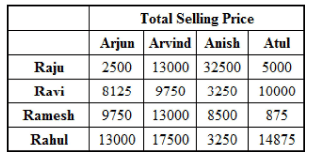The total selling price was more than Rs. 15,000 for 2 teams: Raju-Anish and Rahul-Arvind.
Hence, option 1.

Sample IIFT Data Interpretation Quiz - Question 16

Eight friends Raju, Ravi, Ramesh, Rahul, Arjun, Arvind, Anish and Atul are skilled at making Handicraft items. In order to make money using their skills, they decide to make a certain number of handicraft items and sell them at a Handicraft Expo. They decided to minimize time and maximize their productivity by forming two groups. The people whose names start with the letter ‘R’ form Group 1 and the people whose names start with the letter ‘A’ form Group 2. Each person from Group 1 forms a team each person from Group 2 to produce a certain number of handicraft items of a particular type, the details of which are given in the tables below.
Table 1 gives details of the type of handicraft item produced by each team.Table 2 gives details of the number of handicraft items produced by each team.Table 3 gives the cost price and selling price of one item of each typeThe money (Cost Price and Profit) is shared according to the number of items each one has worked on. For example, if Rahul and Arvind made 175 pots, the total cost price and the profit made by selling those pots would be shared equally.

Q. For how many teams was the ratio of the total cost price to the total selling price less than 0.5?

Detailed Solution for Sample IIFT Data Interpretation Quiz - Question 16

The ratio of cost price to selling price is less than 0.5 for only baskets and plates.
Hence, any team that has made baskets or plates satisfies the given conditions. 4 teams have made baskets and 1 team has made plates i.e. 5 teams satisfy the condition.
Hence, option 3.

Sample IIFT Data Interpretation Quiz - Question 17

Eight friends Raju, Ravi, Ramesh, Rahul, Arjun, Arvind, Anish and Atul are skilled at making Handicraft items. In order to make money using their skills, they decide to make a certain number of handicraft items and sell them at a Handicraft Expo. They decided to minimize time and maximize their productivity by forming two groups. The people whose names start with the letter ‘R’ form Group 1 and the people whose names start with the letter ‘A’ form Group 2. Each person from Group 1 forms a team each person from Group 2 to produce a certain number of handicraft items of a particular type, the details of which are given in the tables below.
Table 1 gives details of the type of handicraft item produced by each team.Table 2 gives details of the number of handicraft items produced by each team.Table 3 gives the cost price and selling price of one item of each typeThe money (Cost Price and Profit) is shared according to the number of items each one has worked on. For example, if Rahul and Arvind made 175 pots, the total cost price and the profit made by selling those pots would be shared equally.

Q. Which of the following statement(s) is/are true?

I. The total profit earned by Atul is Rs. 6,000

II. The total selling price of all the items that Ramesh has worked on is Rs. 32,125

III. The team of Raju and Arjun had the lowest total selling price of the items they had produced.

Detailed Solution for Sample IIFT Data Interpretation Quiz - Question 17

Statement III can be directly verified from the solutions to one of the earlier questions.
The team of Ramesh-Atul has the lowest selling price.
Hence, statement III is false.
Hence, options 2 and 4 can be eliminated.
Since II is present in both the remaining options, you can directly assume it to be true.
Hence, just verify I.

The total profit earned by Atul = (50/2) * 30 + (100/2) x 30 + (25/2 x 20) + (175/2 x 40) = Rs. 6,000 Thus, statement I is true.

Hence, option 1.

Sample IIFT Data Interpretation Quiz - Question 18

Eight friends Raju, Ravi, Ramesh, Rahul, Arjun, Arvind, Anish and Atul are skilled at making Handicraft items. In order to make money using their skills, they decide to make a certain number of handicraft items and sell them at a Handicraft Expo. They decided to minimize time and maximize their productivity by forming two groups. The people whose names start with the letter ‘R’ form Group 1 and the people whose names start with the letter ‘A’ form Group 2. Each person from Group 1 forms a team each person from Group 2 to produce a certain number of handicraft items of a particular type, the details of which are given in the tables below.
Table 1 gives details of the type of handicraft item produced by each team.Table 2 gives details of the number of handicraft items produced by each team.Table 3 gives the cost price and selling price of one item of each typeThe money (Cost Price and Profit) is shared according to the number of items each one has worked on. For example, if Rahul and Arvind made 175 pots, the total cost price and the profit made by selling those pots would be shared equally.

Q. For which type of handicraft was the total number of items produced the highest?

Detailed Solution for Sample IIFT Data Interpretation Quiz - Question 18

The total number of items for each type of handicraft is: Baskets = 125 + 200 + 50 + 50 = 425 Shoes = 75 + 100 + 75 + 100 + 250 = 600 Pots = 25 + 175 + 50 + 100 = 350 Lanterns = 100 + 175 = 275 Hence, option 2

## IIFT Mock Test Series

1 docs|46 tests
 Use Code STAYHOME200 and get INR 200 additional OFF Use Coupon Code
Information about Sample IIFT Data Interpretation Quiz Page
In this test you can find the Exam questions for Sample IIFT Data Interpretation Quiz solved & explained in the simplest way possible. Besides giving Questions and answers for Sample IIFT Data Interpretation Quiz, EduRev gives you an ample number of Online tests for practice

## IIFT Mock Test Series

1 docs|46 tests

### How to Prepare for CAT

Read our guide to prepare for CAT which is created by Toppers & the best Teachers